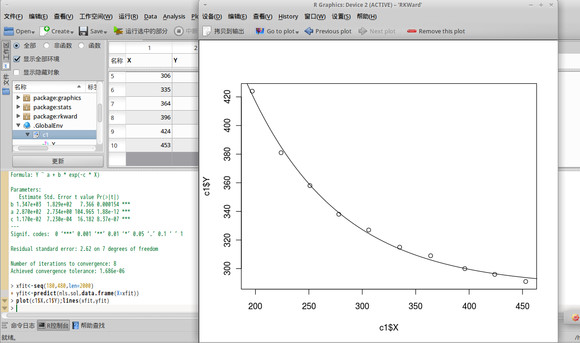# 运用R做数据分析

## 1.什么是R？

R 其实是Rick Becker,John Chambers 和Allan Wilks 开发的 S 语言的一种实现，这三个人都在贝尔实验室工作，他们合作开发了S语言来进行统计的运算，并且开发了商用的SPLUS。关于R的历史，大家可以google。

## 3.R的语法

### 3.1数据结构

• 数值型(numeric): 1,1.2,3.1415926
• 复数型(complex): 1+2i
• 字符型(character): ‘A’/”hello world!”
• 逻辑型(logical): TRUE/FALSE

R中基本的数据对象为下列五类：

1. 向量(vector)
2. 矩阵(matrix)
3. 列表(list)
4. 数据框(data frame)
5. 函数(function)

### 3.2基本类型的操作

X1<-c(......)


X1<-c(1,2,3,4)


y1<-matrix(c(1,2,3,4,5,6,7,8,9),nrow=3,ncol=3,byrow=FALSE)

y2<-matrix(1:9,nrow=3,ncol=3,byrow=FALSE)


x<-list(gender=c('F','M'),grade=c(98,100,90),undergrad=TRUE)## 4.总结

Written on February 9, 2018